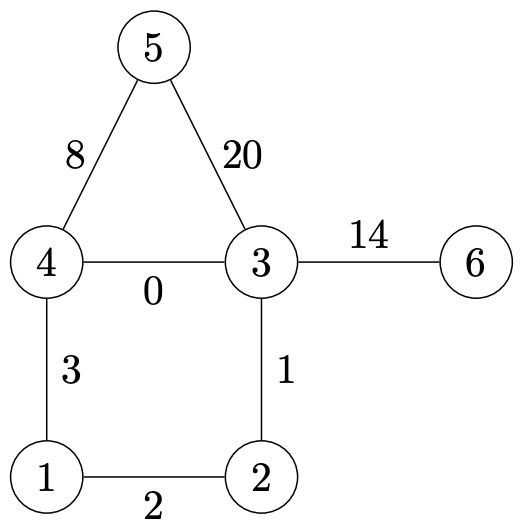시간 제한메모리 제한제출정답맞힌 사람정답 비율
8 초 (추가 시간 없음) 256 MB222100.000%

## 문제

Octagon is in charge of many facilities of strategic importance, such as rocket silos, radars, canteens, veteran offices, uniform warehouses, \ldots It is of utter importance to secure the network of connections between those facilities, but on the other hand, the threat to this network should be minimized. For each bidirectional road linking different facilities, the Attack Threat Coefficient (colloquially called the coefficient) was determined. Johnny, recently employed by the Octagon, even in the middle of the night can compute a subset of roads that minimizes the sum of coefficients and still allows moving between any pair of facilities; we call a road that is in at least one such a subset a key road.

However, nothing can be taken for granted -- coefficients may change. As part of the annual evaluation, the Octagon decided to compute for each road the highest value of $x$ such that when its coefficient is set to $x$ (and all other coefficients are left as they were), this road is a key road. This task was assigned to Johnny, who cannot err in such an important matter.

## 입력

The first line of the input contains two integers $n$  and $m$ ($1 \leq n \leq 100\,000$, $n - 1 \leq m \leq 10^6$), separated by a single space denoting the number of facilities under Octagon's supervision  and the number of bidirectional roads between them, respectively. The facilities are numbered with consecutive natural numbers from $1$ to $n$.

In each of the following $m$ lines there are three integers: $a$, $b$  and $c$ ($1 \leq a, b \leq n$, $a \neq b$, $0 \leq c \leq 10^9$), each separated by a single space, describing a bidirectional road between facilities $a$  and $b$ with coefficient $c$. Any unordered pair $\{a, b \}$ occurs at most once.

Existing roads always allow moving between any two facilities.

## 출력

You should write $m$ lines, one for each road from the input, in the same order in which the roads are given in the input. In the line corresponding to a particular road you should write one natural number -- the maximum value such that after changing the road coefficient to this value (and leaving other coefficients unchanged) this road is a key road. If this value is greater than $10^9$ or can be arbitrarily large, you should write $10^9$.

## 예제 입력 1

6 7
1 2 2
2 3 1
3 4 0
1 4 3
3 5 20
4 5 8
3 6 14


## 예제 출력 1

3
3
3
2
8
20
1000000000


## 힌트

The road network from the example looks as follows: